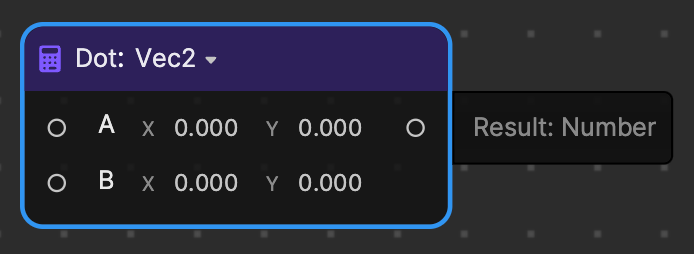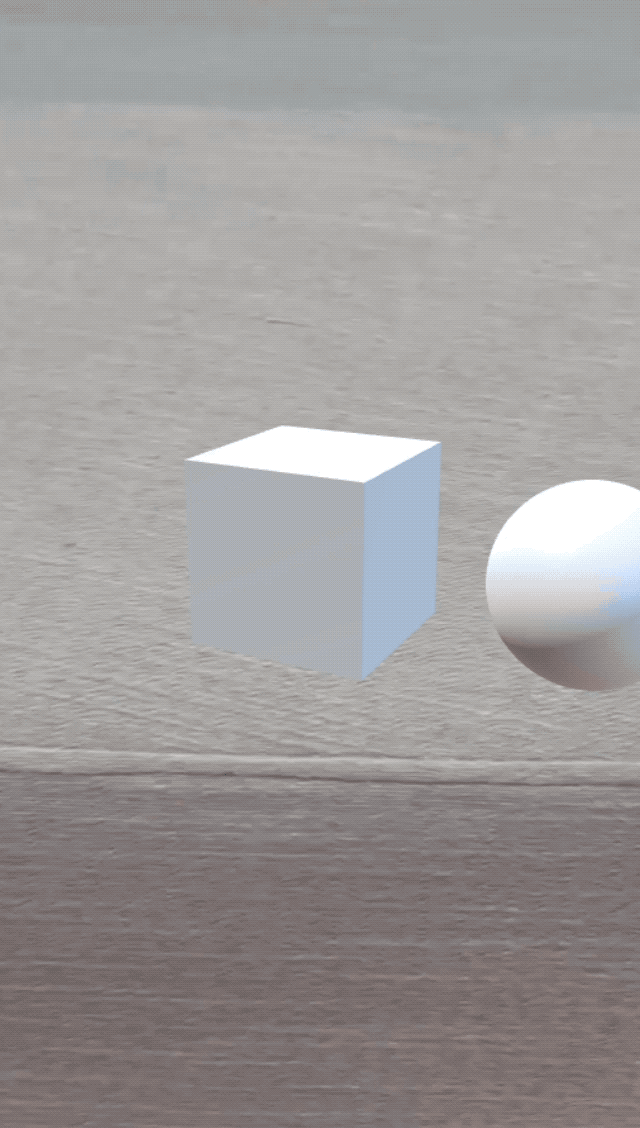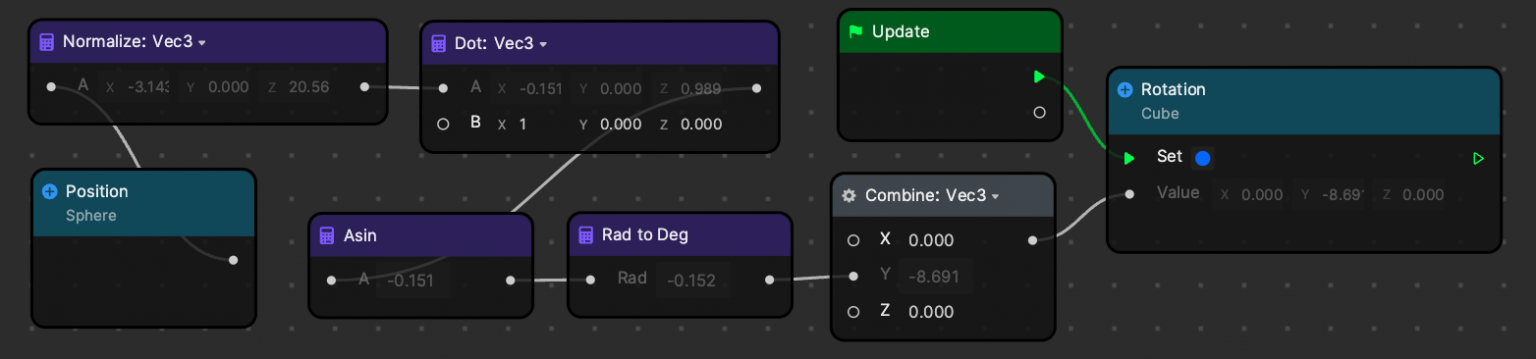Version: 3.5.0

# DotUse Dot to calculate the dot product between two vectors. For example, the dot product of vector A [1, -3, -2] and B [5, -1, 1] is:[1, -3, -2] · [5, -1, 1] = (1 x 5) + (-3 x -1) + (-2 x 1) = 6Supported types: Vec2, Vec3, and Vec4. Dot is in the Math category.

## Input​

NameData TypeDescription
AVec4The first vector.
BVec4The second vector.

## Output​

NameData TypeDescription
ResultVec4The result of the cross product.

## Example​The cube’s rotation is controlled by the position of the sphere. This example uses vector to calculate the angle between sphere position and unit vector along X-Axis. Then it applies this angle for the cube’s rotation.